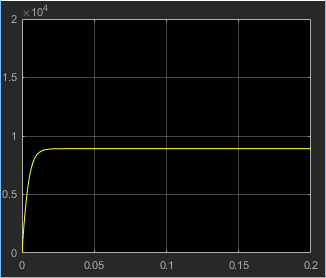## Evaluating Performance of a DC Motor

This example shows how to simulate systems that span electrical and mechanical domains. You learn how to model physical components with Simscape™ blocks, connect them into a realistic model, use Simulink® blocks as well, and then simulate and modify a motor model.

The model is based on a Faulhaber Series 0615 DC-Micromotor. The model uses equivalent circuit parameters for the 1.5V motor to verify manufacturer-quoted no-load speed, no-load current, and stall torque. You can use the model to assess motor performance in a given application by adding the requisite mechanical load model.

### Explore the Model

1. Open the Permanent Magnet DC Motor example model. At the MATLAB® command prompt, enter:

`openExample('simscape/PermanentMagnetDCMotorExample')`

Main Model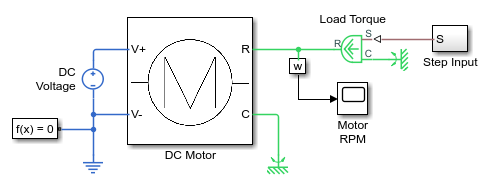The model contains a DC Motor subsystem with two electrical and two mechanical rotational ports.

For improved readability of block diagrams, each Simscape domain uses a distinct default color and line style for the connection lines. In this block diagram, for example, the electrical circuit is indicated by the dark-blue color of the connection lines, while the connection lines between the mechanical rotational ports are light-green. Physical signal lines are brown.

The electrical ports of the motor connect to the electrical circuit, which consists of an Electrical Reference block, representing an electrical ground, and a 1.5 V DC voltage source.

On the mechanical side, a Mechanical Rotational Reference block represents a reference point for the other elements.

The motor load is represented by the block named Load Torque, which is an Ideal Torque Source block. On one side it is connected to a Mechanical Rotational Reference block and on the other side to the motor shaft. The load torque is specified by the Step Input subsystem, connected through a physical signal to the control port S of the Load Torque block.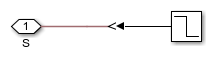The Step Input subsystem contains a regular Simulink Step source, which provides the control signal. A Simulink-PS Converter block converts the control signal into a physical signal and applies it to the control port of the Load Torque block through the Connection Port block S.

The diagram also contains a Solver Configuration block, which is required in any Simscape model. It contains parameters relevant to numerical algorithms for Simscape simulations.

2. Double-click the DC Motor subsystem to open it.

DC Motor Subsystem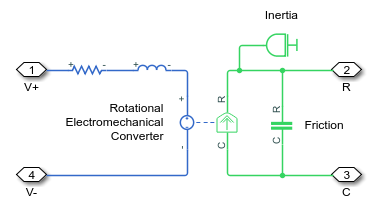The motor consists of an electrical circuit and a mechanical rotational circuit, connected by the Rotational Electromechanical Converter block. The electrical circuit consists of a Resistor block and an Inductor block. It contains two electrical ports, corresponding to the V+ and V- electric terminals of the motor. The mechanical circuit contains a Rotational Friction block, an Inertia block, and two mechanical rotational ports, C and R, corresponding to the motor case and rotor, respectively. Notice how the C and R ports of the Rotational Friction block and the Rotational Electromechanical Converter block are connected to the C and R ports of the motor, to preserve the correct direction of variables in the physical network.

### Run the Model

1. Double-click the Motor RPM scope to open it. During simulation, this window displays the shaft speed as a function of time.

2. Click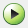to start the simulation. The Simscape solver evaluates the model, calculates the initial conditions, and runs the simulation. This process might take a few seconds. The message in the bottom-left corner of the model window provides the status.

3. Examine the simulation results in the Motor RPM scope window.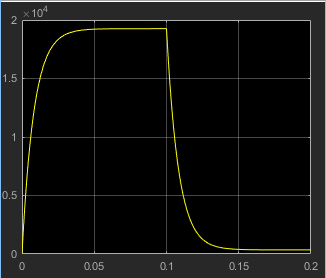For the first 0.1 seconds, the motor has no external load, and the speed builds up to the no-load value. Then at 0.1 seconds, the stall torque is applied as a load to the motor shaft. Zooming in on the Motor RPM scope shows that the model matches the manufacturer parameters for no-load speed and stall torque.

4. The example model also shows how you can use MATLAB code to analyze the simulation results. To plot the current and load torque, click the `Plot` hyperlink in the model annotation. The current shown in the figure matches the manufacturer parameters for no-load current.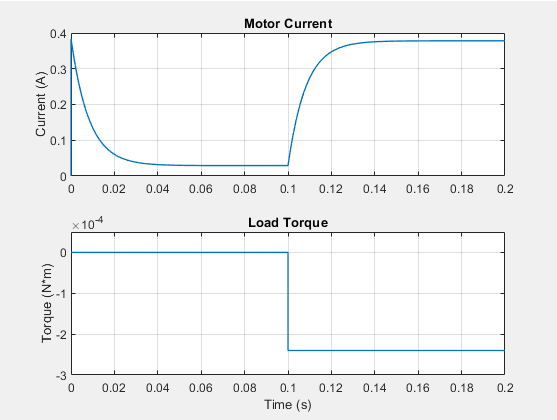### Change the Supply Voltage

Reduce the supply voltage to 1.25 volts (to simulate the battery running down) and vary the load torque to find the maximum torque at this reduced voltage.

1. Double-click the DC Voltage Source block. Set Constant voltage to ```1.25 V```.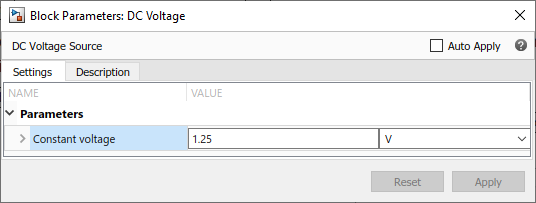2. Run the simulation. Note the effect of reduced voltage on the no-load speed.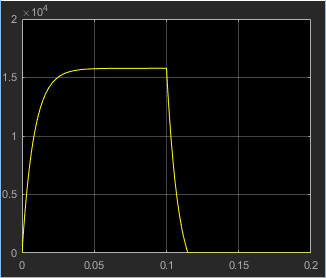3. Try varying the load torque to find the maximum torque at this reduced voltage. Open the Step Input subsystem and double-click the Step source block. Enter different final values for the input signal and rerun the simulation.

The next illustration shows the simulation results for Final value set to `-0.2e-3`, which corresponds to `(1.25/1.5)*0.24mNm`, as the magnitude of the torque-speed curve is proportional to voltage for a DC motor.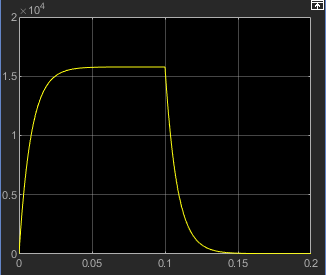Replace the torque source with a simple mechanical load, for example, a fan, for which the torque is defined by `alpha*speed^2`, where `alpha` is `-1e-10` Nm/(rad/s)^2.

1. Delete the Step Input subsystem from the model.

2. In the Simscape block library, open Foundation Library > Mechanical > Mechanical Sensors.

3. Drag the Ideal Rotational Motion Sensor block to the model window.

4. Open Foundation Library > Physical Signals > Functions.

5. Drag the PS Product block and the PS Gain block to the model window.

6. Connect the blocks as shown in the following illustration. To rotate a block, select it and press Ctrl+R.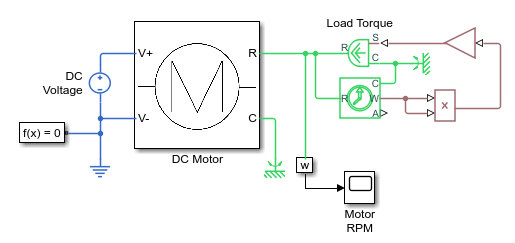7. Double-click the PS Gain block. In the first edit box next to the Gain parameter, enter the value of `-1e-10`. In the second combo box, with the unit drop-down list, type the unit expression `N*m/(rad/s)^2` and click .

8. Run the simulation and assess motor performance with the new load.